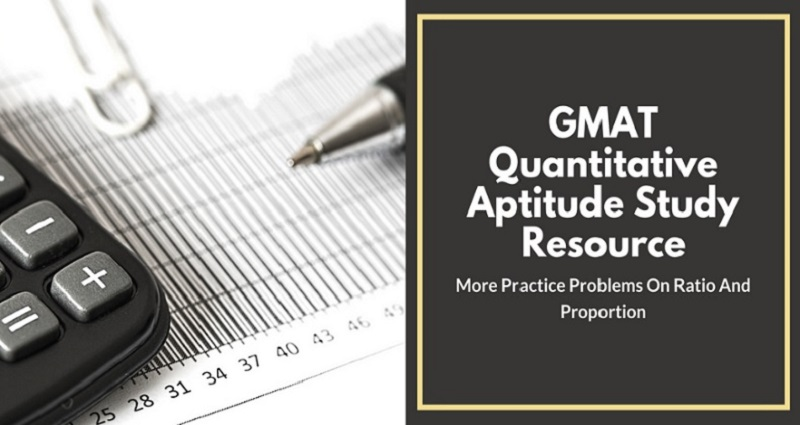X

# GMAT Quantitative Aptitude Study Resource: More Practice Problems On Ratio And Proportion

Aug 28, 2018In one of our previous GMAT QA study resource posts, we discussed a few shortcut tips and practice problems related particularly to the ratio and proportion topic.

Today, in this post, we are going to add to that. Here you go.

Question 3

$432 is divided amongst three workers A, B and C such that 8 times A’s share is equal to 12 times B’s share which is equal to 6 times C’s share. How much did A get? 1.$192

2. $133 3.$144

4. $128 Solution: 8 times A’s share = 12 times B’s share = 6 times C’s share. Note that this is not the same as the ratio of their wages being 8 : 12 : 6 In this case, find out the L.C.M of 8, 12 and 6 and divide the L.C.M by each of the above numbers to get the ratio of their respective shares. The L.C.M of 8, 12 and 6 is 24. Therefore, the ratio A:B:C :: 24/8 : 24/12 : 24/6 => A : B : C :: 3 : 2 : 4 The sum of the total wages = 3x + 2x + 4x = 432 => 9x = 432 or x = 48. Hence, A who gets 3x will get 3 * 48 =$144.

Answer: c) $144. Question 4 In a mixture of 45 liters, the ratio of sugar solution to salt solution is 1:2. What is the amount of sugar solution to be added if the ratio has to be 2:1? 1. 45 liters 2. 50 liters 3. 55 liters 4. 40 liters Solution: Number of liters of sugar solution in the mixture = (1/(1+2)) *45 = 15 litres. So, 45-15 = 30 liters of salt solution is present in it. Let the quantity of sugar solution to be added be x litres. Setting up the proportion, sugar solution / salt solution = (15+x)/30 = 2/1 or, x = 45. Therefore, 45 liters of sugar solution has to be added to bring it to the ratio 2:1. Answer: a) 45 liters. Question 5 Two numbers are in the ratio 3 : 4. If the sum of numbers is 63, find the numbers. 1. 55, 80 2. 25, 67 3. 27, 36 4. 40, 50 Solution: Sum of the terms of the ratio = 3 + 4 = 7 Sum of numbers = 63 Therefore, first number = 3/7 × 63 = 27 Second number = 4/7 × 63 = 36 Therefore, the two numbers are 27 and 36. Answer: c) 27, 36 Question 6 A, B and C enter into a partnership by investing$3600, $4400 and$2800. A is a working partner and gets a fourth of the profit for his services and the remaining profit is divided amongst the three in the rate of their investments. What is the amount of profit that B gets if A gets a total of $8000? 1. 4888.88 2. 9333.33 3. 4000 4. 3666.66 Solution: Let x be the profit. Their investment ratio = 3600: 4400: 2800 = 9 : 11 : 7 A's profit of$8000 = (1/4 * x) + 1/3 (3/4*x) = 1/2 * x

x = $16,000 Therefore B's profit = 11/27(3/4 * 16000) =$4888.88

Question 7

A, B and C play cricket. A's runs are to B's runs and B's runs are to C's as 3:2. If altogether, they score 342 runs. How many runs did A make?

1. 162

2. 108

3. 72

4. None of these

Solution:

A:B = 3:2 = 9:6;

B:C = 3:2 = 6:4 (making B equal)

Therefore, A:B:C = 9:6:4

Therefore, the runs made by A = (9/19) X 342 = 162.